# Variable FIR Decimation

Polyphase FIR decimation with tunable decimation factor

Since R2023a

•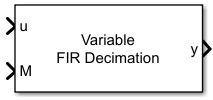Libraries:
DSP System Toolbox / Filtering / Multirate Filters

## Description

The Variable FIR Decimation block performs an efficient polyphase FIR decimation with a tunable decimation factor. You can update the decimation factor and the filter coefficients in the block dialog box or through an input port while the simulation is running.

Conceptually, the FIR decimator (as shown in the schematic) consists of an anti-aliasing FIR filter followed by a downsampler. To design an FIR anti-aliasing filter, use the `designMultirateFIR` function.

The FIR filter filters the data in each channel of the input using a direct-form FIR filter. The downsampler that follows downsamples each channel of filtered data by taking every M-th sample and discarding the M – 1 samples that follow. M is the value of the decimation factor. The resulting discrete-time signal has a sample rate that is 1/M times the original sample rate.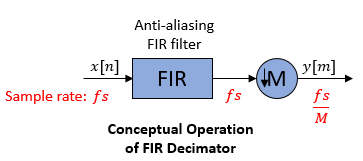Note that the actual block algorithm implements a direct-form FIR polyphase structure, an efficient equivalent of the combined system depicted in the diagram. For more details, see Algorithms.

The block supports C and C++ code generation.

## Examples

expand all

Since R2023b

Decimate a sinusoidal signal whose frame size varies during simulation. The Variable FIR Decimation block determines the frame size of the decimated output based on the value of the Specification parameter.

Specify Decimation Factor

Open and inspect the SpecifyDecimationFactor.slx model. The Specification parameter in the Variable FIR Decimation block dialog box is set to `Decimation factor`. In this mode, you specify the decimation factor in the block dialog box or through an input port. When the input frame size varies during simulation, the block maintains this decimation factor and varies the output frame size so that output frame size equals the ratio of input frame size and decimation factor.

The Variable FIR Decimation block outputs a variable-size signal.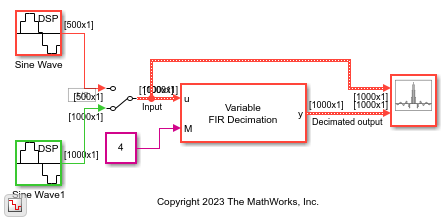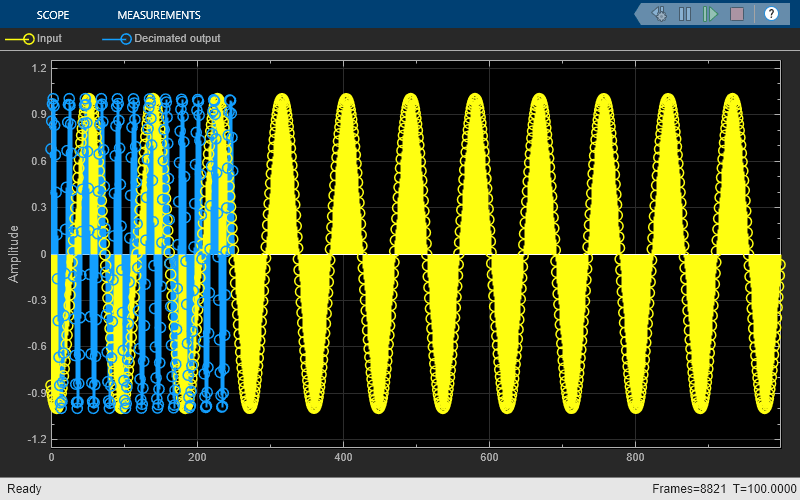Specify Output Frame Length

Open and inspect the SpecifyOutputFrameLength.slx model. The Specification parameter in the Variable FIR Decimation block dialog box is set to `Output frame length`. In this mode, you specify the output frame length in the block dialog box. During simulation, when the input frame size varies, the block varies the decimation factor in order to maintain the frame length of the output signal. The output frame size equals the ratio of input frame size and decimation factor.

The Variable FIR Decimation block outputs a fixed-size signal.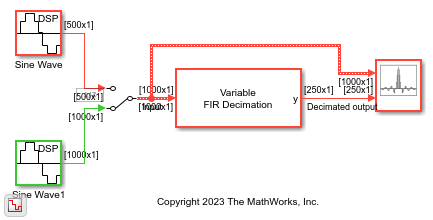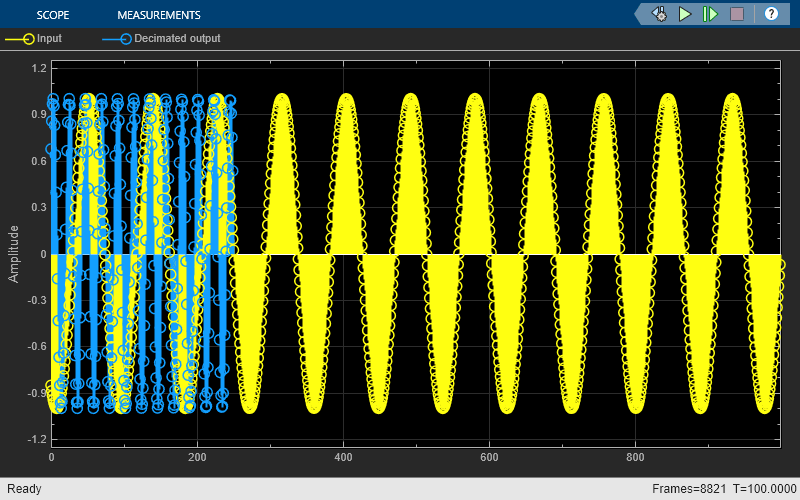Decimate a sinusoidal signal by varying the decimation factor using the Variable FIR Decimation block. You can vary the decimation factor in the block dialog box or through an input port while the simulation is running.

Open the model. The input is a sinusoidal signal with a frequency of 500 Hz, sample time of 1/44100 s, and contains 100 samples per frame. Pass this signal through the Variable FIR Decimation block. The Maximum decimation factor parameter in the block is 24. The decimation factor that you input through the port is 4.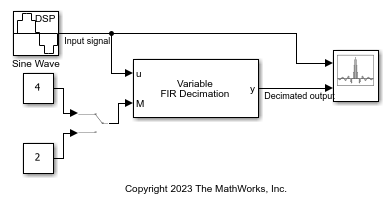Run the model. The Array Plot block shows the input signal and the decimated output on the display.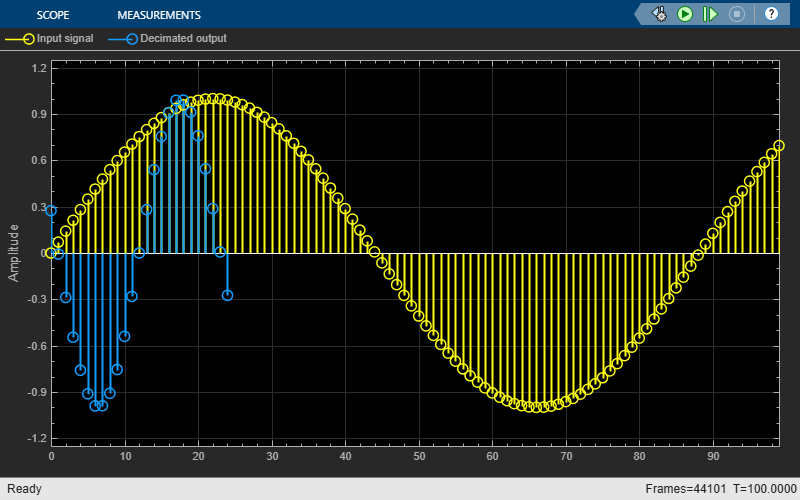While the simulation is running, change the decimation factor to 2 by clicking the Manual Switch. The span of the decimated output updates in the Array Plot display. You can change the decimation factor to any value that is an integer factor of the maximum decimation factor of 24.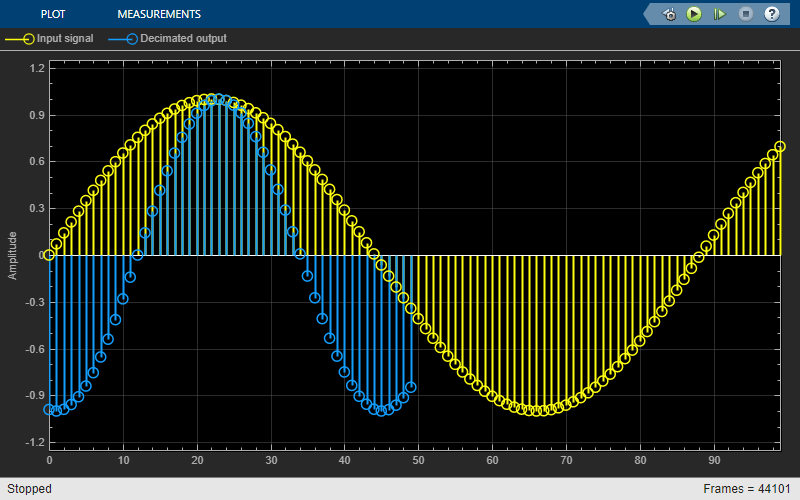If you specify the decimation factor in the block dialog box, you can tune the Decimation factor parameter in the block dialog box while the simulation is running and the block updates the decimated output accordingly.

Decimate a sinusoidal signal using the Variable FIR Decimation block. You can vary the filter coefficients in the block dialog or through an input port while the simulation is running.

Open the model. The input is a sinusoidal signal with a frequency of 500 Hz, sample time of 1/44100 s, and contains 100 samples per frame. Pass this signal through the Variable FIR Decimation block. The Maximum decimation factor parameter in the Variable FIR Decimation block is set to 24. Specify the decimation factor and the filter coefficients through the M and the coeffs ports, respectively. The decimation factor is 4 and the filter coefficients are generated using the `designMultirateFIR(1,24)` function. This function generates an effective anti-aliasing lowpass filter with a normalized cutoff frequency no greater than 1/24.

You can vary the filter coefficients using the Manual Switch.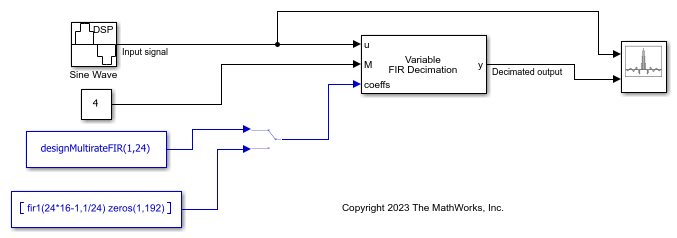Run the model. The Array Plot block shows the input signal and the decimated output in the display.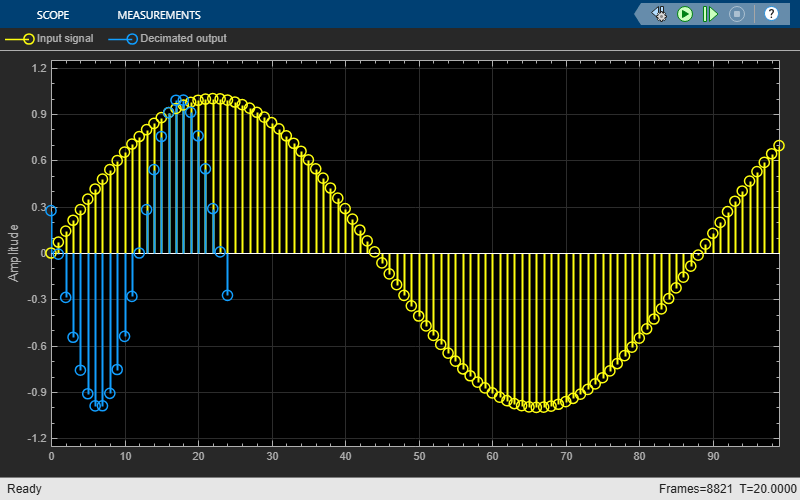While the simulation is running, change the filter coefficients by clicking the Manual Switch. On the second branch, the `fir1` function generates the coefficients of a lowpass filter that has a similar passband frequency response and the same number of coefficients as the first filter. Note that you cannot change the number of filter coefficients while the simulation is running.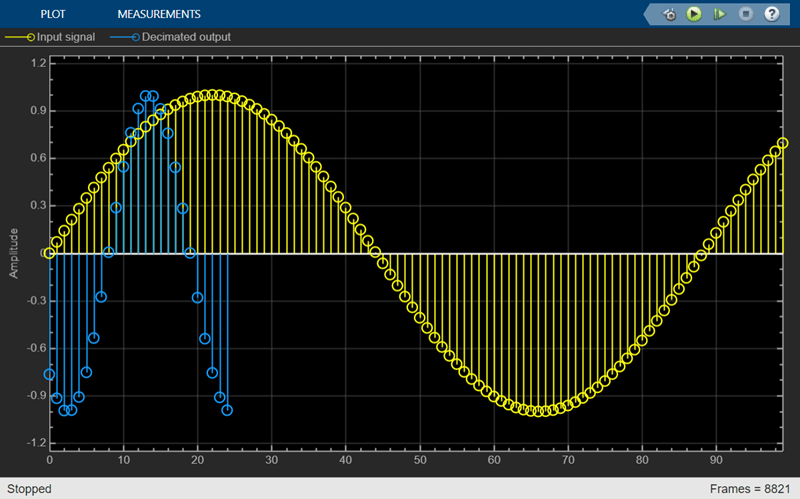## Ports

### Input

expand all

Specify the data input as a vector or a matrix of size P-by-Q. The block treats each column of the input signal as a separate channel. If the input is a two-dimensional signal, the first dimension represents the channel length (or frame size) and the second dimension represents the number of channels. If the input is a one-dimensional signal, then the block interprets it as a single channel.

The block accepts variable-size input signals, that is, you can change the size of each input channel during simulation, but you cannot change the number of channels.

When you set Specification to ```Output frame length```, the input frame length P must be an integer multiple of the output frame length Po. (since R2023b)

Data Types: `single` | `double`
Complex Number Support: Yes

Specify the decimation factor M as a positive integer that is an integer factor of the maximum decimation factor. For example, if you set Maximum decimation factor to 24, then the possible values for decimation factor are 1, 2, 3, 4, 6, 8, 12, and 24.

You can tune the decimation factor while the simulation is running. For an example, see Decimate Sinusoidal Signal with Tunable Decimation Factor.

#### Dependencies

To enable this port:

• Set Specification to ```Decimation factor```. (since R2023b)

• Select the Specify decimation factor from input port parameter.

Data Types: `single` | `double` | `int8` | `int16` | `int32` | `int64` | `uint8` | `uint16` | `uint32` | `uint64`

Specify the lowpass FIR filter coefficients in descending powers of z as a vector.

The transfer function H(z) of the FIR filter is given by:

`$H\left(z\right)={b}_{0}+{b}_{1}{z}^{-1}+...+{b}_{N}{z}^{-N}$`

You can generate the FIR filter coefficient vector, b = [b0, b1, …, bN], using one of the DSP System Toolbox™ filter design functions such as `designMultirateFIR`, `firnyquist`, `firhalfband`, `firgr`, or `firceqrip`.

Compute the filter coefficients based on the maximum decimation factor Mmax instead of the decimation factor M. For example, if Mmax is 24 and M is 8, and you used `designMultirateFIR` as the design function, compute the filter coefficients using `designMultirateFIR(1,24)` instead of `designMultirateFIR(1,8)`.

To act as an effective anti-aliasing filter, the coefficients usually correspond to a lowpass filter with a normalized cutoff frequency no greater than 1/Mmax. To design such a filter, use the `designMultirateFIR` function.

The block internally initializes all filter states to zero.

You can change the filter coefficients during simulation but the number of filter coefficients must remain constant. For an example, see Specify Filter Coefficients Through Input Port.

#### Dependencies

To enable this port, set the Coefficient source parameter to `Input port`.

Data Types: `single` | `double`
Complex Number Support: Yes

### Output

expand all

Decimated output, returned as a vector or a matrix.

When you set the Specification parameter to `Decimation factor` (since R2023b), the size of the output signal is same as the size of the input signal. However, the block computes a maximum of `ceil`(P/M)-by-Q output samples, where P is the input frame size, Q is the number of input channels, and M is the decimation factor.

If the input is a variable-size signal, the block outputs a variable-size signal so as to maintain the decimation factor.

When you set the Specification parameter to ```Output frame length```, the size of the output signal is Po-by-Q, where Po is the value you specify in the Output frame length parameter. (since R2023b)

If the input is a variable-size signal, the block varies the decimation factor accordingly and outputs a fixed-size signal. (since R2023b)

The complexity of the output signal depends on the complexity of the input signal and the complexity of the filter coefficients. See this table for more details.

Input SignalFilter CoefficientsOutput Signal
RealComplexComplex
RealRealReal
ComplexComplexComplex
ComplexRealComplex

Data Types: `single` | `double`
Complex Number Support: Yes

## Parameters

expand all

Since R2023b

Specify the mode in which you control the decimation factor.

When you set Specification to:

• `Decimation factor` –– The block maintains the decimation factor that you specify. If the input signal frame length changes during simulation (variable-size input signal), the output frame length changes in a way so as to maintain the decimation factor M.

A variable-size input generates a variable-size output.

• `Output frame length` –– The block maintains the output frame length that you specify. If the input signal frame length changes during simulation (variable-size input signal), the decimation factor changes in a way so as to maintain the output frame length Po.

A variable-size input generates a fixed-size output.

Specify the maximum decimation factor Mmax as a positive integer. The decimation factor you specify through the input port M must be a factor of the value you specify in this parameter.

When you set the Specification parameter to ```Output frame length```, the block treats the input frame length as the maximum decimation factor Mmax. (since R2023b)

#### Dependencies

To enable this port, set the Specification parameter to ```Decimation factor```. (since R2023b)

When you select this parameter, you can specify the decimation factor through the input port M. When you clear this parameter, you can specify the decimation factor in the block dialog box through the Decimation factor parameter.

#### Dependencies

To enable this parameter, set the Specification parameter to ```Decimation factor```. (since R2023b)

Specify the decimation factor M as a positive integer that is an integer factor of the maximum decimation factor Mmax. For example, if you set Maximum decimation factor to 24, then the possible values for Decimation factor are 1, 2, 3, 4, 6, 8, 12, and 24.

You can tune the decimation factor while the simulation is running.

When you set the Specification parameter to ```Output frame length```, the block treats the ratio of the input frame length P to the output frame length Po as the decimation factor M, that is, M = P/Po. (since R2023b)

Tunable: Yes

#### Dependencies

To enable this parameter:

• Set the Specification parameter to ```Decimation factor```. (since R2023b)

• Clear the Specify decimation factor from input port parameter.

Since R2023b

Specify the output frame length Po as a positive integer. In this mode, the block maintains the frame length of the output signal at the value you specify in this parameter. If the input signal frame length changes during simulation (variable-size input signal), the decimation factor of the block changes in a way so as to maintain the output frame length.

The input frame length must be an integer multiple of the output frame length.

The value you specify in this parameter determines the current decimation factor M. For more details, see the Decimation factor parameter.

#### Dependencies

To enable this parameter, set the Specification parameter to `Output frame length`.

Specify the FIR filter coefficient source as one of the following:

• `Auto` –– The block designs an FIR decimator using the decimation factor in the Decimation factor parameter. The `designMultirateFIR` function designs the filter and returns the coefficients used by the block.

• `Dialog parameter` –– Specify the filter coefficients through the Prototype filter coefficients parameter in the block dialog box.

• `Input port` –– Specify the filter coefficients through the coeffs input port.

Specify the lowpass FIR filter coefficients in descending powers of z as a vector. By default, `designMultirateFIR(1,24)` computes the filter coefficients with the maximum decimation factor Mmax of 24.

The transfer function H(z) of the FIR filter is given by:

`$H\left(z\right)={b}_{0}+{b}_{1}{z}^{-1}+...+{b}_{N}{z}^{-N}$`

You can generate the FIR filter coefficient vector, b = [b0, b1, …, bN], using one of the DSP System Toolbox filter design functions such as `designMultirateFIR`, `firnyquist`, `firhalfband`, `firgr`, or `firceqrip`.

Compute the filter coefficients based on the maximum decimation factor Mmax instead of the decimation factor M. For example, if Mmax is 24 and M is 8, and you used `designMultirateFIR` as the design function, compute the filter coefficients using `designMultirateFIR(1,24)` instead of `designMultirateFIR(1,8)`.

To act as an effective anti-aliasing filter, the coefficients usually correspond to a lowpass filter with a normalized cutoff frequency no greater than 1/Mmax. To design such a filter, use the `designMultirateFIR` function.

The block internally initializes all filter states to zero.

Tunable: Yes

#### Dependencies

To enable this parameter, set the Coefficient source parameter to `Dialog parameter`.

Click this button to open the dynamic filter visualizer and display the magnitude response of the variable FIR decimation filter. The response is based on the parameters you select in the block dialog box. To update the magnitude response while the dynamic filter visualizer is running, modify the parameters in the dialog box and click .

You cannot view the filter response when you set the Specification parameter to ```Output frame length``` (since R2023b) or the Coefficient source parameter to `Input port`. To view the filter response, set the Specification parameter to `Decimation factor` (since R2023b) and the Coefficient source parameter to `Auto` or ```Dialog parameter```.You can configure the plot settings and the signal measurements from the interface of the visualizer.

On the Plot tab, the Configuration section allows you to modify the plot settings. Click Magnitude Phase to display the magnitude and phase response of the filter.On the Measurements tab, you can measure the signal statistics, place data cursors, and display the peak values of the selected signal.For more details on the dynamic filter visualizer interface and its tools, see `dsp.DynamicFilterVisualizer`.

Specify the type of simulation to run. You can set this parameter to:

• `Code generation`: Simulate model using generated C code. The first time you run a simulation, Simulink® generates C code for the block. The C code is reused for subsequent simulations, as long as the model does not change. This option requires additional startup time but provides faster simulation speed than ```Interpreted execution```.

• `Interpreted execution`: Simulate model using the MATLAB®  interpreter. This option shortens startup time but has slower simulation speed than `Code generation`.

## Block Characteristics

 Data Types `double` | `single` Direct Feedthrough `no` Multidimensional Signals `no` Variable-Size Signals `yes` Zero-Crossing Detection `no`

expand all

## Algorithms

The FIR decimation filter is implemented efficiently using a polyphase structure. For more details on polyphase filters, see Polyphase Subfilters.

To derive the polyphase structure, start with the transfer function of the FIR filter:

`$H\left(z\right)={b}_{0}+{b}_{1}{z}^{-1}+...+{b}_{N}{z}^{-N}$`

N+1 is the length of the FIR filter.

You can rearrange this equation as follows:

`$H\left(z\right)=\begin{array}{c}\left({b}_{0}+{b}_{{M}_{\text{max}}}{z}^{-{M}_{\text{max}}}+{b}_{2{M}_{\text{max}}}{z}^{-2{M}_{\text{max}}}+...+{b}_{N-{M}_{\text{max}}+1}{z}^{-\left(N-{M}_{\text{max}}+1\right)}\right)+\\ {z}^{-1}\left({b}_{1}+{b}_{{M}_{\text{max}}+1}{z}^{-{M}_{\text{max}}}+{b}_{2{M}_{\text{max}}+1}{z}^{-2{M}_{\text{max}}}+...+{b}_{N-{M}_{\text{max}}+2}{z}^{-\left(N-{M}_{\text{max}}+1\right)}\right)+\\ \begin{array}{c}⋮\\ {z}^{-\left({M}_{\text{max}}-1\right)}\left({b}_{{M}_{\text{max}}-1}+{b}_{2{M}_{\text{max}}-1}{z}^{-{M}_{\text{max}}}+{b}_{3{M}_{\text{max}}-1}{z}^{-2{M}_{\text{max}}}+...+{b}_{N}{z}^{-\left(N-{M}_{\text{max}}+1\right)}\right)\end{array}\end{array}$`

Mmax is the number of polyphase components, and its value equals the maximum decimation factor.

You can write H(z) as:

`$H\left(z\right)={E}_{0}\left({z}^{{M}_{\text{max}}}\right)+{z}^{-1}{E}_{1}\left({z}^{{M}_{\text{max}}}\right)+...+{z}^{-\left({M}_{\text{max}}-1\right)}{E}_{{M}_{\text{max}}-1}\left({z}^{{M}_{\text{max}}}\right)$`

E0(zMmax), E1(zMmax), ..., EMmax-1(zMmax) are the polyphase components of the FIR filter H(z).

During simulation, the algorithm reconstructs the filter H(z) based on the current decimation factor M.

Rewriting H(z) in terms of the decimation factor M.

`$H\left(z\right)=r{E}_{0}\left({z}^{M}\right)+{z}^{-1}r{E}_{r}\left({z}^{M}\right)+...+{z}^{-\left(M-1\right)}r{E}_{\left(M-1\right)r}\left({z}^{M}\right)$`

where, r = Mmax/M.

Conceptually, the FIR decimation filter contains a lowpass FIR filter followed by a downsampler.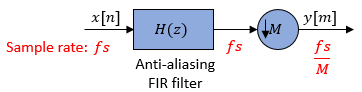Replace H(z) with its polyphase representation.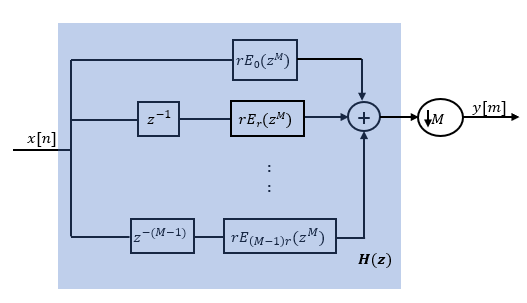Here is the multirate noble identity for decimation.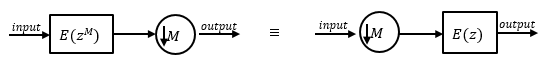Applying the noble identity for decimation moves the downsampling operation to before the filtering operation. This move enables you to filter the signal at a lower rate.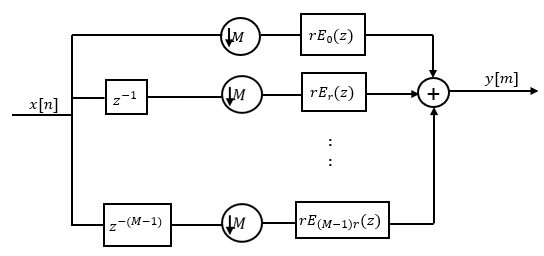You can replace the delays and the decimation factor at the input with a commutator switch. The switch starts on the first branch 0 and moves in the counterclockwise direction as shown in this diagram. The accumulator at the output receives the processed input samples from each branch of the polyphase structure and accumulates these processed samples until the switch goes to branch 0. When the switch goes to branch 0, the accumulator outputs the accumulated value.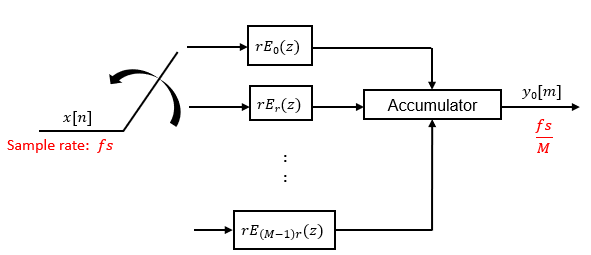When the first input sample is delivered, the switch feeds this input to the branch 0 and the decimator computes the first output value. As more input samples come in, the switch moves in the counter clockwise direction through branches (M−1)r, (M−2)r, and all the way up to branch 0, delivering one sample at a time to each branch. When the switch comes to branch 0, the decimator outputs the next set of output values. This process continues as data keeps coming in. Every time the switch comes to the branch 0, the decimator outputs y[m]. The decimator effectively outputs one sample for every M samples it receives. Hence the sample rate at the output of the FIR decimation filter is fs/M.

 Orfanidis, Sophocles J. Introduction to Signal Processing. Upper Saddle River, NJ: Prentice-Hall, 1996.